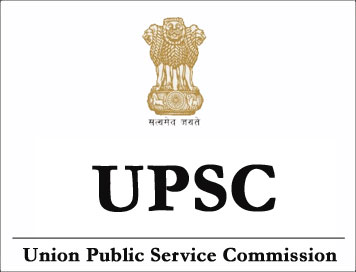NEW!

# (Syllabus) Indian Statistical Service Examination (ISS) - STATISTICS - II## STATISTICS-II

1. Linear Models:  Theory of linear estimation. Gauss-Markoff setup. Least square estimators. Use of ginverse. analysis of one-way and two way classified data-fixed, mixed and random effect models. Tests for regression coefficients.

2. Estimation: Characteristics of good estimator. Estimation methods of maximum likelihood, minimum chi-square, moments and least squares. Optimal properties of maximum likelihood estimators. Minimum variance unbiased estimators. Minimum variance bound estimators. Cramer-Rao inequality. Bhattacharya bounds. Sufficient estimator. factorisation theorem. Complete statistics. Rao-Blackwell theorem. Confidence interval estimation. Optimum confidence bounds. Resampling, Bootstrap and Jacknife.

3. Hypotheses testing and Statistical Quality Control: (a) Hypothesis testing: Simple and composite hypothesis. Two kinds of error. Critical region. Different types of critical regions and similar regions. Power function. Mostpowerful and uniformly most powerful tests. Neyman-Pearson fundamental lemma. Unbiased test. Randomised test. Likelihood ratio test. Wald’s SPRT, OC and ASN functions. Elements of decision and game theory. b) Statistical Quality Control: Control Charts for variable and attributes. Acceptance Sampling by attributes-Single, double, multiple and sequential Sampling plans; Concepts of AOQL and ATI; Acceptance Sampling by variables-use of Dodge-Romig and other tables.

4. Multivariate Analysis: Multivariate normal distribution. Estimation of mean Vector and covariance matrix. Distribution of Hotelling’s T2-statistic, Mahalanobis’s D2-statistic, and their use in testing. Partial and multiple correlation coefficients in samples from a multivariate normal population. Wishart’s distribution, its reproductive and other properties. Wilk’s criterion. Discriminant function. Principal components. Canonical variates and correlations.